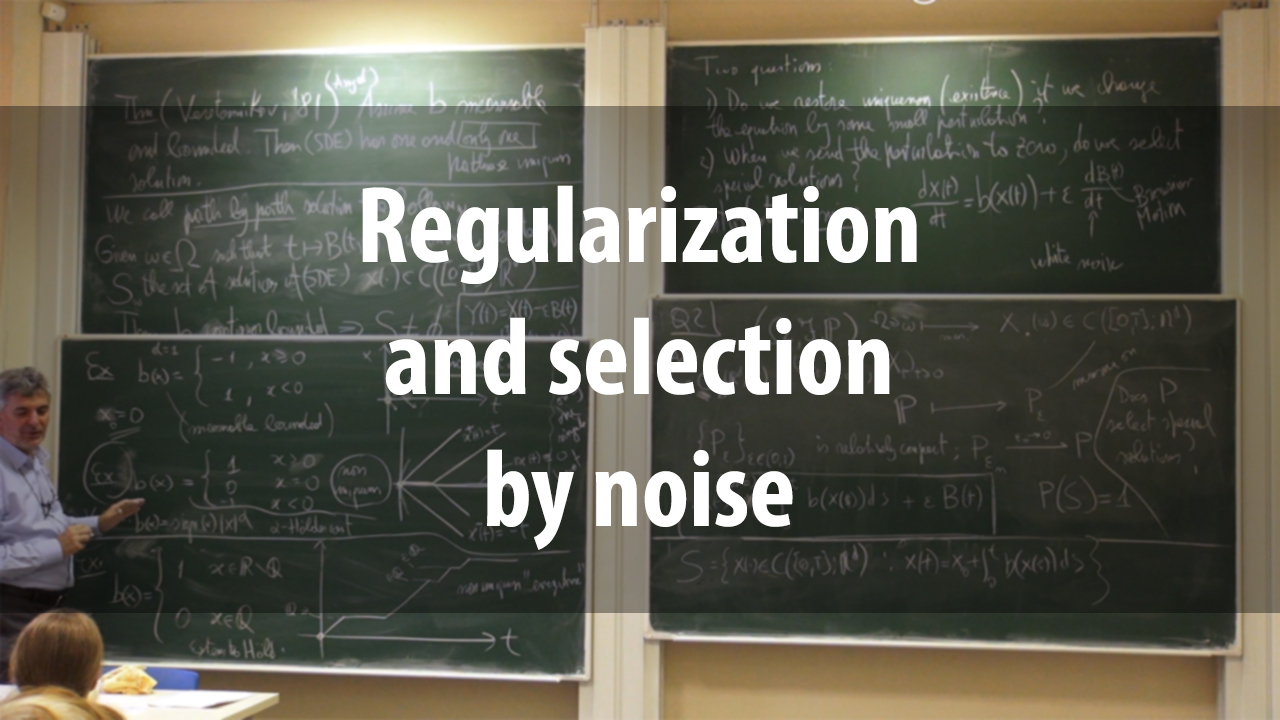# Regularization and selection by noise

Курс
Предмет:
Лектор:The most classical properties we ask on a differential equation are existence and uniqueness. Existence has its own philosophy, going for instance in the direction of enlarging the meaning of the equation and thus the class of potential solutions; even if some of the topics of this minicourse intersect the question of existence, it will not be the main task. Uniqueness is our main concern. For certain equation and classes of solutions it is not fulfilled, or it is an open problem; examples will be recalled, mainly for ordinary differential equations for sake of simplicity, but also for certain partial differential equations.

Probability has several links with the question of uniqueness. In the minicourse we shall discuss three of them: restoring uniqueness by noise; selecting special solutions of a deterministic equation by means of a zero-noise limit; detecting the existence of intrinsically stochastic solutions of certain deterministic equations. The reason why noise in able to restore uniqueness for equations with very singular behavior is understood in a number of cases; one could even say that there is nowadays a theory. However, it is still a very active field of research, especially for nonlinear partial differential equations. The zero-noise limit is, on the other side, almost not understood, except for one-dimensional differential equations, which will be our main example. It is a striking open problem even for certain simple 2D examples that will be shown. Its potentiality is therefore still not completely explored.

The topic of intrinsic stochasticity is related: we look for examples where uniqueness fails for a deterministic equation and also the zero-noise limit does not identify a single special solution, but some truly stochastic process emerges as interesting solution of the deterministic equation.

The minicourse is based on the Inventiones paper and there are some early drafts of lecture notes.

2